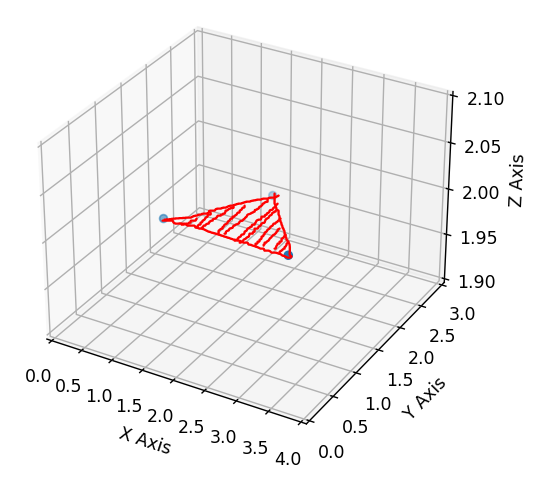# Best Way to Fill 3D Scatter Points? (MatPlotLib, Py 3.10)

I have the following `Python 3.10` script to generate a simple 3D Scatter Plot with `MatPlotLib` , according to the MatPlotLib 3D Scatter tutorial:

``````import matplotlib.pyplot as plt

fig = plt.figure()

X = [1, 3, 2]
Y = [1, 1, 2]
Z = [2, 2, 2]

ax.scatter(X, Y, Z)

ax.set_xlim(0, 4)
ax.set_ylim(0, 3)
ax.set_zlim(1.9, 2.1)

ax.set_xlabel('X Axis')
ax.set_ylabel('Y Axis')
ax.set_zlabel('Z Axis')

plt.show()
``````

The script above works as intended, and I get the correct output:However, I was wondering whether it would be possible to connect & fill the scatter points, to create a “face” of sorts, and to provide a color for it. In this case, it would be as such:I looked around the other `MatPlotLib` tutorials, notably the ones for the 3D Surface Colormap plot and the 3D Surface Solid Color plot, however it’s confusing to me how they are implemented.

From what I could gather by attempting to run the examples, the Z-axis data set is a multi-dimensional array (the interperter threw up an error if I simply supplied a singular list or np array to it). I don’t really understand why the Z-axis data set needs to be a multi-dimensional array, as I would imagine that the actual data colors are supplied via the `color` argument to the `scatter` function call.

Is what I’m trying to do even possible with `MatPlotLib`? Is there a better way to approach this?

Thanks for reading my post, any guidance is appreciated!